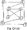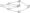# Analysis and Design of Algorithms July 2009

Note: Answer any FIVE full questions.

1 a. Compare adjacency matrix and adjacency list methods of representing graphs. Give examples.

b. Write two separate algorithms to compute the indegree and outdegree of a directed, connected graph, which is represented as adjacency list.

2 a. Define the following asymptotic notations: 0,(H), Q.

b. Prove the following, using limit theory: i) log (n!)=fi(nlog nj   ii) Vl0n2+7n+3 =@(x)

c.   Write an iterative/recursive algorithm to find an and determine the time taken by your algorithm (apply brute-force method).

3 a. Show the steps in sorting the list using quicksort: 60, 50, 20, 80, 10, 90, 30, 70, 40.

b.  Using divide-and-conquer approach, write an algorithm for Quicksort and derive the best, worst, and average complexity of the same.

4 a. Write DFS and BFS trees for the following graph:b.  Write an algorithm or C/C++ program to find the path between any two given vertices u and v of an undirected graph G – {V, E} based on DFS. You must print all the vertices appearing between u and v. Note that the path need not be the shortest.

5 a. What do you understand by Topological sort? Give its applications. Apply source removal method to find the topological order of the following graph:b.  An efficient algorithm to generate all permutations of a positive integer n is by using Johnson-Trotter algorithm. Show the algorithm and all the intermediate steps for n = 3. Suggest the best data structure to implement this algorithm.

6 a. Show how would you sort the following list using Heapsort algorithm:

34, 12, 89, 34, 21, 12, 64, 10

b.  Explain the Horspool algorithm by taking an appropriate example.

c.   Devise a dynamic programming based algorithm for finding the all-pairs shortest path of a given connected, directed graph G. Trace your algorithm for the below given cost matrix.

 1 2 3 4 1 0 00 3 6 2 2 0 00 4 3 co 7 0 1 4 00 00 00 0

7 a. Let G – {V, E} be an undirected, connected graph. Write the Prim’s algorithm that finds the minimum spanning tree based on greedy technique. Give an example of a 5 vertex graph to illustrate its working.

b.  Apply backtracking to solve the following instance of sum-of-subset problem:

S – {1, 5,2, 7} with d = 8

8 a. Solve the following problem using Branch and Bound technique:

Job Persons

 Jobl Job2 Job3 Job4 A 9 2 7 8 B 6 4 3 7 C 5 8 1 8 D 7 6 9 4

B  Discuss the approximation algorithms for NP-hard problems.